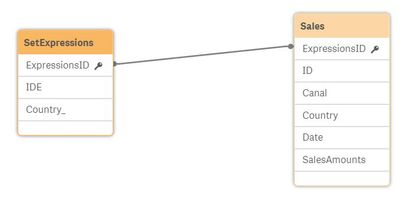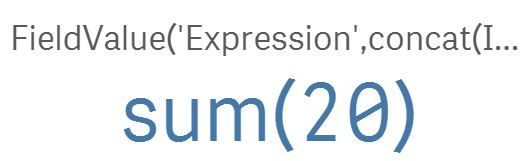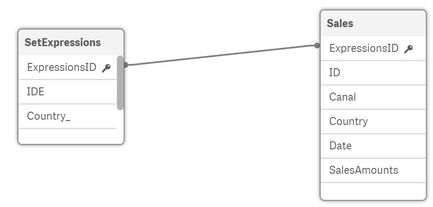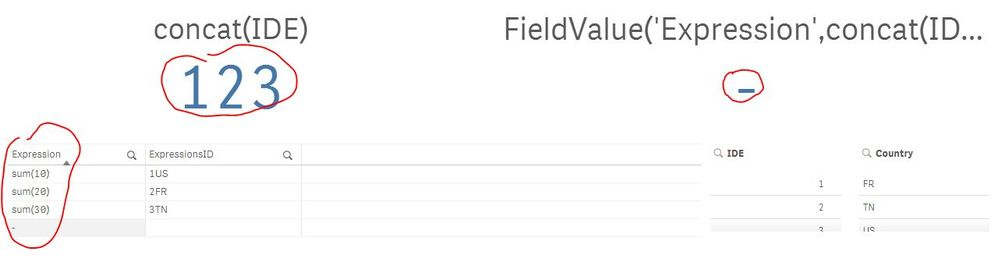Announcements
Qlik Analytics Tour 2020 Online. Begins August 10th. Register Today
cancel
Showing results for
Did you mean:
HighlightedContributor III

## Scenario Planning Model - Applying different input variables to each category within a field

Hi,

TLDR:

I needed to do something similar to the person in this post: https://community.qlik.com/t5/Qlik-Sense-App-Development/InputField-and-InputSum-in-QlikSense/td-p/4....  What I am trying to understand is how do you create an input variable for each category (because they require different calculations) without using nested if statements. I dont think nested if statements will work because the combination of input variables will require 50+ nested if statements.

Background:

In order to accomplish this, I created a basic scenario planning table in haste without spending too much time on thinking about how to model it properly. The goal of the model is  to analyze cost savings based on inputs. It is a simple model, you have one variable (Level) and seven categories within Level (1-7). Using the variable extension, seven different sliders are used (because they have different calculations), so that you can adjust each category accordingly. Below is sample code. As you can see,  all the calculations are in the front end, uses nested IF formulas, its really messy and I am sure there is a much cleaner way of doing this. Not to mention, this code is repeated, for each business, this is just for one business.

if([ELT Level]=1 and count({\$<SBU={"US"},[Is Manager]={"No"}>}[Full Name])<=0, (sum({\$<SBU={"US"},[Is Manager]={"No"}>}Median)+(median({1<[Is Manager]={"No"}>}[Median])*vUSnumemp1)),
if([ELT Level]=1,(sum({\$<SBU={"US"},[Is Manager]={"No"}>}Median)+(median({\$<SBU={"US"},[Is Manager]={"No"}>}[Median])*vUSnumemp1)),
if([ELT Level]=2 AND count({\$<SBU={"US"},[Is Manager]={"No"}>}[Full Name])<=0, (sum({\$<SBU={"US"},[Is Manager]={"No"}>}Median)+(median({1<[Is Manager]={"No"}>}[Median])*vUSnumemp2)),
if([ELT Level]=2,(sum({\$<SBU={"US"},[Is Manager]={"No"}>}Median)+(median({\$<SBU={"US"},[Is Manager]={"No"}>}[Median])*vUSnumemp2)),
if([ELT Level]=3 and count({\$<SBU={"US"},[Is Manager]={"No"}>}[Full Name])<=0, (sum({\$<SBU={"US"},[Is Manager]={"No"}>}Median)+(median({1<[Is Manager]={"No"}>}[Median])*vUSnumemp3)),
if([ELT Level]=3,(sum({\$<SBU={"US"},[Is Manager]={"No"}>}Median)+(median({\$<SBU={"US"},[Is Manager]={"No"}>}[Median])*vUSnumemp3)),
if([ELT Level]=4 and count({\$<SBU={"US"},[Is Manager]={"No"}>}[Full Name])<=0, (sum({\$<SBU={"US"},[Is Manager]={"No"}>}Median)+(median({1<[Is Manager]={"No"}>}[Median])*vUSnumemp4)),
if([ELT Level]=4,(sum({\$<SBU={"US"},[Is Manager]={"No"}>}Median)+(median({\$<SBU={"US"},[Is Manager]={"No"}>}[Median])*vUSnumemp4)),
if([ELT Level]=5 and count({\$<SBU={"US"},[Is Manager]={"No"}>}[Full Name])<=0, (sum({\$<SBU={"US"},[Is Manager]={"No"}>}Median)+(median({1<[Is Manager]={"No"}>}[Median])*vUSnumemp5)),
if([ELT Level]=5,(sum({\$<SBU={"US"},[Is Manager]={"No"}>}Median)+(median({\$<SBU={"US"},[Is Manager]={"No"}>}[Median])*vUSnumemp5)),
if([ELT Level]=6 and count({\$<SBU={"US"},[Is Manager]={"No"}>}[Full Name])<=0, (sum({\$<SBU={"US"},[Is Manager]={"No"}>}Median)+(median({1<[Is Manager]={"No"}>}[Median])*vUSnumemp6)),
if([ELT Level]=6,(sum({\$<SBU={"US"},[Is Manager]={"No"}>}Median)+(median({\$<SBU={"US"},[Is Manager]={"No"}>}[Median])*vUSnumemp6)),
if([ELT Level]=7 and count({\$<SBU={"US"},[Is Manager]={"No"}>}[Full Name])<=0, (sum({\$<SBU={"US"},[Is Manager]={"No"}>}Median)+(median({1<[Is Manager]={"No"}>}[Median])*vUSnumemp7)),
if([ELT Level]=7,(sum({\$<SBU={"US"},[Is Manager]={"No"}>}Median)+(median({\$<SBU={"US"},[Is Manager]={"No"}>}[Median])*vUSnumemp7)),
if([ELT Level]=8 and count({\$<SBU={"US"},[Is Manager]={"No"}>}[Full Name])<=0, (sum({\$<SBU={"US"},[Is Manager]={"No"}>}Median)+(median({1<[Is Manager]={"No"}>}[Median])*vUSnumemp8)),
if([ELT Level]=8,(sum({\$<SBU={"US"},[Is Manager]={"No"}>}Median)+(median({\$<SBU={"US"},[Is Manager]={"No"}>}[Median])*vUSnumemp8)),
0
))))))))))))))))

Now I need to make the model more complex by adding another variable (city) that has 15 categories, but the functionality still needs to remain the same, adjust the input by level AND city  which if I use this same approach, would require like ~50+ nested if formulas. I suspect this will decrease performance, but also, simply bothers me as it is ugly code.

So my question is, how do I do this in a more concise, clean manner. One thought I had was creating another table,  which lists the cost of every combination (i.e., level by city), and than applying the variable to that table. However, I am still unsure on how to get around using the nested if statements so that each input is correctly applied to the right combination, which is really what I am trying to understand. I tried using set analysis, but it applies the input variable to all of the categories, rather than just one.

Thanks!

8 Replies
HighlightedPartner

## Re: Scenario Planning Model - Applying different input variables to each category within a field

What these variables contain

vUSnumemp1 (2,3,4,5,6,7,8) ?

Why don't you load a seperate field that contains the levels like

``````LevelsField:

Level

1

2

3

4

5

7

];
``````

use getcurrentselect() function to get the list of values selected in the dashboard, if you select value 1 on from field LevelsField

getcurrentselection() : LevelsField:1

if you select year = 2019 for example

getcurrentselection() returns : LevelsField:1, Year:2019

So use wildmatch to focus one the fields that you want to use for calculation with wildmatch()

Wildmatch(getcurrentselection(), "LevelsField:*") : returns everything that contains LevelsField

Now, you can use substring methods to extract the values selected in that string like mid(..)

In case for example Wildmatch(getcurrentselection(), "LevelsField:*") rerurns LevelsField:1

mid(Wildmatch(getcurrentselection(), "LevelsField:*"),13,1) will return 1

which is the value you can use to filter you calculation in set expression

the same for Country, let say you have Country field representing country with two characters

mid(Wildmatch(getcurrentselection(), "Country:*"),8,2) will return US

``````Sum({<LevelsField = {mid(Wildmatch(getcurrentselection(), "LevelsField:*"),13,1)},

Country = {mid(Wildmatch(getcurrentselection(), "Country:*"),8,2)}>}Values)``````

I am writing the logic without any trials so the goal is to avoid using if(), and translate the logical thingking into a set analysis expresssion, because this way even if you have 120 values selected you don't care, because the expression simply filters the field based on the selected values on Dashboard.

Hope that helps 🙂

HighlightedContributor III

## Re: Scenario Planning Model - Applying different input variables to each category within a field

Thanks for the response Lazurens! But Im not sure if this will fit my needs. In the dashboard, there are no selections made for Level. To clarify, a person adjusts the sliders for each level, which in turn adjusts the table (or chart) for each level. I have attached screenshots of the tool to help demonstrate.

HighlightedContributor III

## Re: Scenario Planning Model - Applying different input variables to each category within a field

bump
HighlightedPartner

## Re: Scenario Planning Model - Applying different input variables to each category within a field

I will suggest the following idea, and excuse me if I did not provide some well developed example I just present the concept here .

The idea is to create a table that contains the formulas that you want to compute based on the sliders of the simulation

this table also contains all the possible individual formulas, each row is linked to dimensions

the selections on the dimensions will lead only one row in the expressions table, as soon as you have got this result the problem is solved and the user selections led to the desired formulas that should be calculated without using the if() statement.``````Sales:
ID, Canal, Country, Date, SalesAmounts, ExpressionsID
1, A, US, 01/1/2018, 300,1US
2, B, FR, 03/3/2018, 1000,2FR
3, A, FR, 30/6/2018, 2000,
4, C, TN, 05/6/2019, 5000,3TN
];

SetExpressions:
IDE, Country_, Expression,ExpressionsID
1, US, sum(10),1US
2, FR, sum(20),2FR
3, TN, sum(30),3TN
];``````

This is a simple example, table sales is linked to many formulas {Sum(10), Sum(20), Sum(30)}, each expression is linked the appropriate case in the sales table with keys (1US, 2FR, 3TN) you can automate the key creation easily with concat() afterwards, I want you to focus on the idea.

In the Dashboard now, I will disply the desired field:As you can See, as soon as you filter by country there is only one possible key in the field "IDE" that is linked to the desired formula for country France, the only thing we need to do now, is get that expression

``FieldValue('Expression',concat(IDE))``

concat(IDE): will return the possible values of the field [IDE] which should be only one value, then with the interrecord function FieldValue() we get the text of the formula from the field ExpressionNow you can use this concept to design the linkage between your dimensions and the expressions that you want to calculate and based on those selections you get the desired expressions, for the cases that you want to display 0, just force the links to end up into 0 for certain selections and you will never need an if() 🙂

Of course you need to put in plain text your expressions : Sum({<SetAnalaysis>}Field)

I hope this helps.

Best Regards,

HighlightedPartner

## Re: Scenario Planning Model - Applying different input variables to each category within a field

I have made a simple example to demonstrate my idea of how approach the problem of having different formulas for different selections.

First, I created a table that contains individual formulas for each case, second I would link each formula (each row) to the corresponding selection(s) for example I have three countries (US, FR, TN) and for each one of them I have a different calculation, I create a unique key (US is linked to the first formula, FR is linked to the second, and TN is linked to the third)

This way I translate the logical problem into a schematic representation that could computed automatically using interrecord functions to build all the combinations you have.

``````Sales:
ID, Canal, Country, Date, SalesAmounts, ExpressionsID
1, A, US, 01/1/2018, 300,1US
2, B, FR, 03/3/2018, 1000,2FR
3, A, FR, 30/6/2018, 2000,
4, C, TN, 05/6/2019, 5000,3TN
];

SetExpressions:
IDE, Country_, Expression,ExpressionsID
1, US, sum(10),1US
2, FR, sum(20),2FR
3, TN, sum(30),3TN
];``````

Now I have SetExpression table linked to each case in the sales table, so I can display the one formula that corresponds the selected country.Initially, I have all possible selection (1,2 and 3) when there is no country selected

concat(IDE): returns possible values of the field ID Expression

Fieldvalue('Expression', concat(IDE)): returns the exression itself of the possible value

So as soon as we have only one possible value, the KPI could be calculated automaticallybase on the selection.As you can see now, I select the country (FR), the IDE would have only the expression of ID 2, so the KPI could execute that expressionYou can build upon this concept, and add more combinations, for example if you want to add male/female, then you should create a unique key that concatenates Expression ID, Country, and Gender as soon as you select country only nothing happens because you still have more possible values for males and females, and when you select male for example the possible values of ID expression will be reduced to only one possible value and you are done.

As I said this is a simple example, I made very fast to demonstrate the concept, and I hope it would help you.

From performance point of view, no IF is needed at all, and you can even go beyound and add flags to the Expression table and use set expression to control more the set of expressions you have and maybe ignore the ones you dislike to compute.

Thanks,

Mohamed

HighlightedContributor III

## Re: Scenario Planning Model - Applying different input variables to each category within a field

Thanks Mohamed! I think this is getting me in the right direction, but I am still not fully understanding, how do you incorporate a slider so that it adjusts on the row level for a table, keeping in mind that the table must remain static.

Using your example, if we have a table with all of the countries listed, in this case, there are three, how can i create a slider for each so that it adjusts the row accordingly. Keeping in mind, the table must remain static, meaning, that all the countries must be listed at all times, there is no filtering. If the table were to be dynamic, i.e., selecting a country, which displays the country in the table and accordingly the associated formula, i think your solution would work, but in my case I need the table to remain the same, just the content in the cells to update based on the input of the slider.

HighlightedContributor III

## Re: Scenario Planning Model - Applying different input variables to each category within a field

so the "solution" I have come up with in the mean time is to use pick match, as I hear that is better for performance. However, I have a total combination of about ~5000 inputs, so unclear whether performance will remain with such a long pick/match formula. Additionally, writing that formula is going to suck. Perhaps I need to change the user design of the dashboard to reduce the number of inputs

HighlightedPartner

## Re: Scenario Planning Model - Applying different input variables to each category within a field

Exactely, I don't think a slider would be a useful UI component for an optimized User experience as you have more than 100 option to deal with because it's not easy to slide over 5000 options.
I suggest to stick basics, simple design and use field selector, that way you can type the values that you search of, and you can even add some logic in the back end to display the values that you need to display.
I also advice you to finish building the logic first not worry so much about the presentation of the dashboard, as soon as you are comfortable with the logic and have it tested and validated, move on and optimize the design.